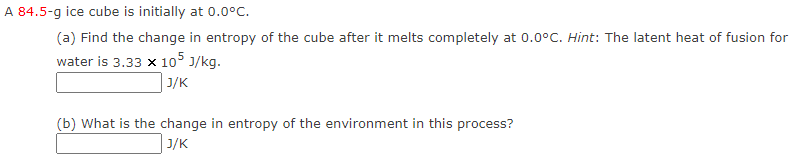Home / Expert Answers / Physics / 7-84-5-mathrm-g-ice-cube-is-initially-at-0-0-circ-mathrm-c-a-find-the-change-pa635

# (Solved): 7 $$84.5-\mathrm{g}$$ ice cube is initially at $$0.0^{\circ} \mathrm{C}$$. (a) Find the change ...

7$$84.5-\mathrm{g}$$ ice cube is initially at $$0.0^{\circ} \mathrm{C}$$. (a) Find the change in entropy of the cube after it melts completely at $$0.0^{\circ} \mathrm{C}$$. Hint: The latent heat of fusion for water is $$3.33 \times 10^{5} \mathrm{~J} / \mathrm{kg}$$. $\mathrm{J} / \mathrm{K}$ (b) What is the change in entropy of the environment in this process? $\mathrm{J} / \mathrm{K}$

We have an Answer from Expert